Next: Partial Waves Up: Scattering Theory Previous: Born Approximation

# Born Expansion

As we have seen, quantum scattering theory requires the solution of the integral equation (10.29),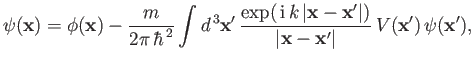(10.45)

where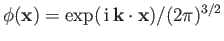is the incident wavefunction, and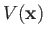the scattering potential. An obvious approach, in the weak-scattering limit, is to solve the preceding equation via a series of successive approximations. That is,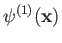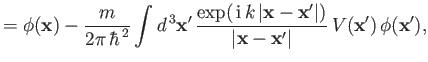(10.46)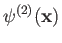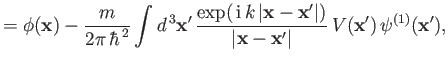(10.47)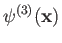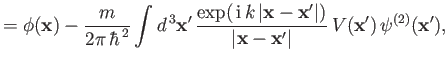(10.48)

and so on. Assuming thatis only non-negligible relatively close to the origin, and taking the limit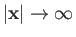, we find that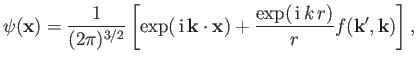(10.49)

where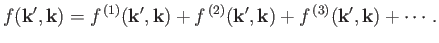(10.50)

The first two terms in the previous series, which is generally known as the Born expansion, are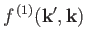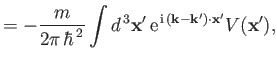(10.51)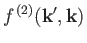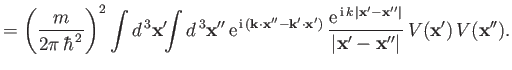(10.52)

Of course, we recognize Expression (10.51) as that produced by the Born approximation discussed in the preceding section. In other words, the Born approximation essentially involves truncating the Born expansion after its first term. Incidentally, it can be proved that the Born expansion converges for all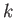(for a spherically symmetric scattering potential) provided; a)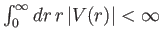; b)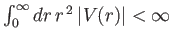; and; c)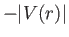is too weak to form a bound state . Furthermore, the criterion for convergence becomes less stringent at high.Next: Partial Waves Up: Scattering Theory Previous: Born Approximation
Richard Fitzpatrick 2016-01-22